# Part 3: Functional Mapping | Further Functions and Relations

Are you struggling with functions and relations? Don't worry, in this guide, we'll give you the run-down you need on functional mapping to get you through Year 11 Maths Advanced.Do you struggle wrapping your head around functional mapping? You’re not alone! In this article, we will be introducing the concepts you need to understand to ace further functions and relations for Year 11 Maths Advanced.

This post will be dedicated to the study of functions, their properties and how they interact when subjected to a range of operations.

## Year 11 Advanced Mathematics: Further Functions and Relations

Understanding relations and functions play a crucial role in many Year 11/12 topics.

It is extremely important for students to recognise the difference between relations and functions especially for future topics such as introductory calculus and curve sketching.

## NESA Syllabus Outcomes

NESA has provided the following outcomes for this topic:

### F1.2: Introduction to Functions

• define and use a function and a relation as mappings between sets, and as a rule or a formula
that defines one variable quantity in terms of another
– define a relation as any set of ordered pairs $$(𝑥, 𝑦)$$ of real numbers
– understand the formal definition of a function as a set of ordered pairs $$(𝑥, 𝑦)$$ of real numbers such that no two ordered pairs have the same first component (or $$𝑥$$-component)
• use function notation, domain and range, independent and dependent variables (ACMMM023)
– understand and use interval notation as a way of representing domain and range, eg $$[4, \infty)$$
• understand the concept of the graph of a function (ACMMM024)
• identify types of functions and relations on a given domain, using a variety of methods
– know what is meant by one-to-one, one-to-many, many-to-one and many-to-many
– use the vertical line test to identify a function
– determine if a function is one-to-one (ACMSM094)
• define odd and even functions algebraically and recognise their geometric properties
• define the sum, difference, product and quotient of functions and consider their domains and ranges where possible

## Assumed Knowledge for Further Functions and Relations

Students should be familiar with graphing basic curves and understanding their equations such as the straight lines, parabolas and circles.

Each point on these curves contain a $$x$$ and $$y$$ coordinate which we will be using in our introduction to relations and functions. Hence, familiarising yourself with a good understanding of graphs and curves is recommended before going through this post.

Students should also be familiar with working with the number line and sketching inequalities onto it.

## Relations

A relation is a set of ordered pairs $$(x,y)$$ where $$x$$ and $$y$$ are real numbers.

“Ordered” pairs means that the order of the $$x$$ and $$y$$ coordinates matter, i.e. $$(3,4)$$ is different to $$(4,3)$$.

Example of relations:

• The set of points $${(1,1),(1,5),(2,4),(3,3)}$$ forms a relation.
• The set of points satisfying the equation $$y=x-1$$ forms a relation. If we plot these points onto a graph, we get a straight line.
• The set of points satisfying the equation $$x^2+y^2=1$$ forms a relation. If we plot these points onto a graph, we get the unit circle.

## Classifying and Mapping Relations

We can use an arrow diagram to represent the relation of mapping one set of points to another set of points. To do this, we list the $$x$$-coordinates into the first set $$X$$ and the $$y$$-coordinates into the second set $$Y$$. Then we draw an arrow from $$x$$ to $$y$$ for each pair of $$(x,y)$$.

Consider the set of points $${(1,1),(1,5),(2,4),(3,3)}$$ from our previous example. We can visualise this relation in the arrow diagram below.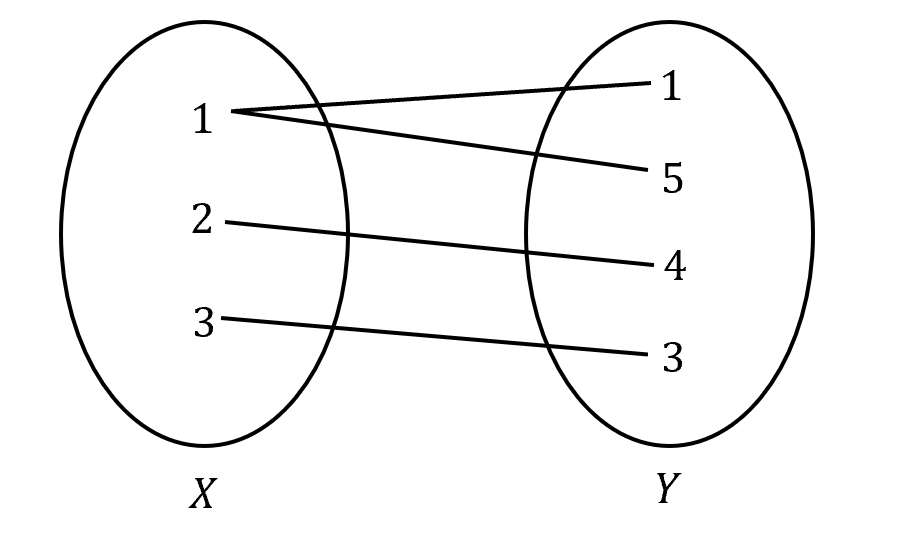This relation is known as a “one-to-many” relation since the first element of set $$X$$, $$1$$, is mapped to more than one element in set $$Y$$, which are $$1$$ and $$5$$.

There are four types of relation mapping which we describe in the table below:

 Type of Mapping Arrow Diagram Description One-to-one Each element of set $$X$$ is mapped to only one element of set $$Y$$Each element of set $$Y$$ is mapped from at most one element of set $$X$$ Many-to-one All elements in set $$X$$ are mapped to at most one element in set $$Y$$.There exists an element in set $$Y$$ which has more than one element mapped to it from set $$X$$. One-to-many There exists an element in set $$X$$ which is mapped to more than one element in set $$Y$$All elements of set $$Y$$ is mapped from at most one element of set $$X$$ Many-to-many There exists an element in set $$X$$ which is mapped to more than one element in set $$Y$$.There exists an element in set $$Y$$ which is mapped from more than one element of set $$X$$.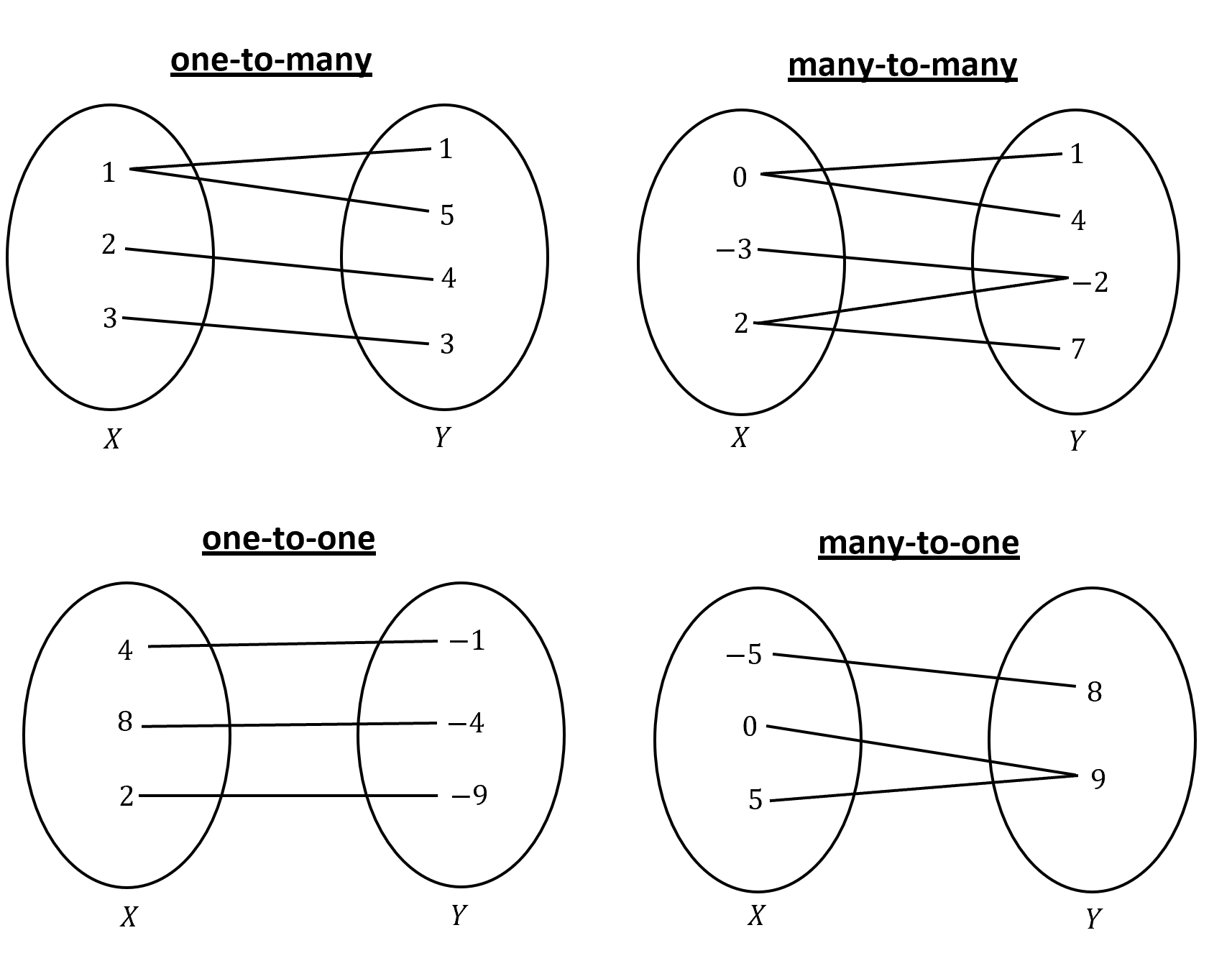## Functions

A function is a set of ordered pair $$(x,y)$$ where every pair has a unique $$x$$ component. This means a function is a relation between two variables where each value of $$x$$ has only one corresponding value for $$y$$. Hence, a function can be a “one-to-one” or “many-to-one” relation however, not all relations are functions.

We can determine whether a relation is a function by drawing vertical lines on the graph of its curve.

• If there is only one point of intersection of a vertical line with the curve, then this verifies that there is only one y-value for every $$x$$-value. Hence, the curve is a function, otherwise the curve is not a function.
• This test is known as the vertical line test.

Example:

Let’s investigate the relations $$x^2+y^2=1$$ and $$y=x^2-1$$ to determine whether they are functions.

Solution:

First, we can draw both curves as shown below.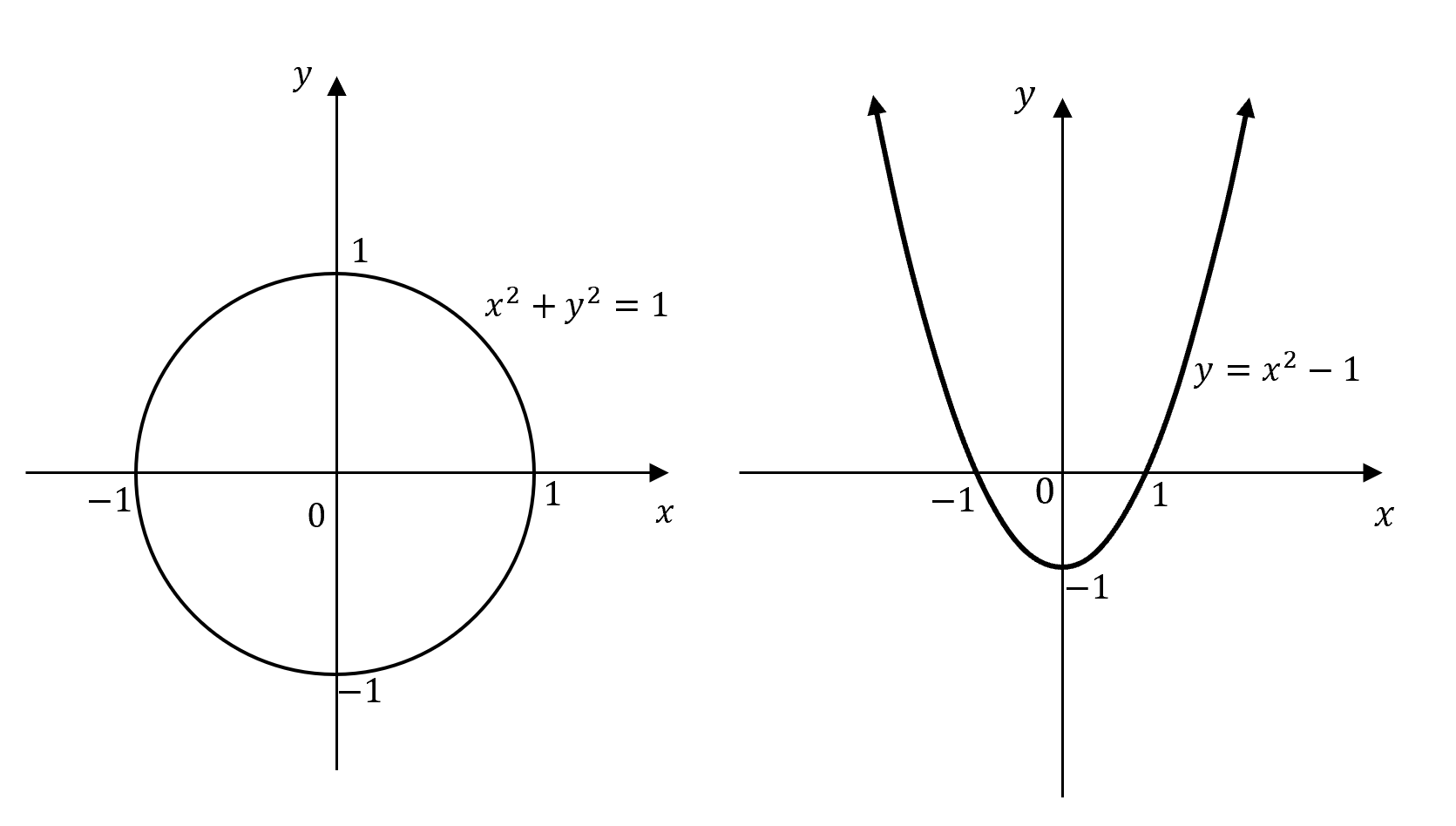Draw any vertical line, say $$x=0.5$$, through the graphs of the curves.

The vertical line intersects the circle at two points whereas it will only intersect the parabola at one point. Therefore, we can say the circle $$x^2+y^2=1$$ is not a function and the parabola $$y=x^2-1$$ is a function because there can only be one point of intersection for the vertical line test.

## Function Notation

Now that we understand what functions are, we can use a special notation to represent them, called function notation. A function can be perceived as a machine that takes inputs and converts it into another form as an output.

Consider the function from our previous example $$y=x^2-1$$.

• We can interpret the function as a machine that inputs $$x$$ values and converts it into outputs of $$y$$ values. In our example, the machine will take in $$x$$-value, square it, and then subtract $$1$$ from it.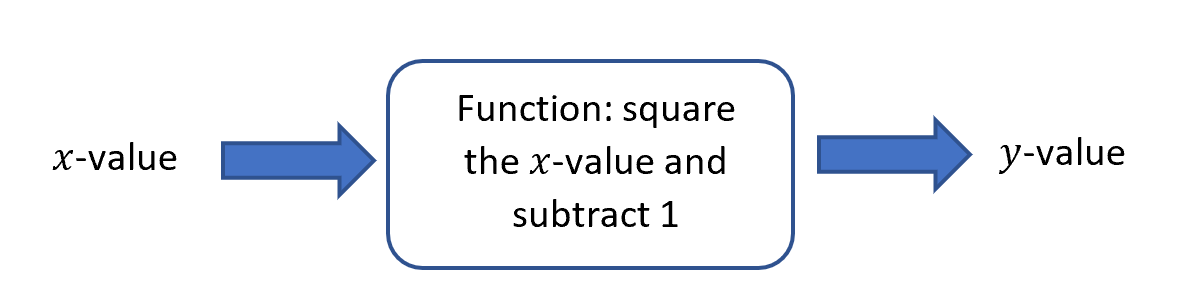• Using function notation, we can rewrite the equation of the curve as $$y=f(x)=x^2-1$$ or simply $$f(x)=x^2-1$$.
• For example, when $$x$$ has a value of $$2$$: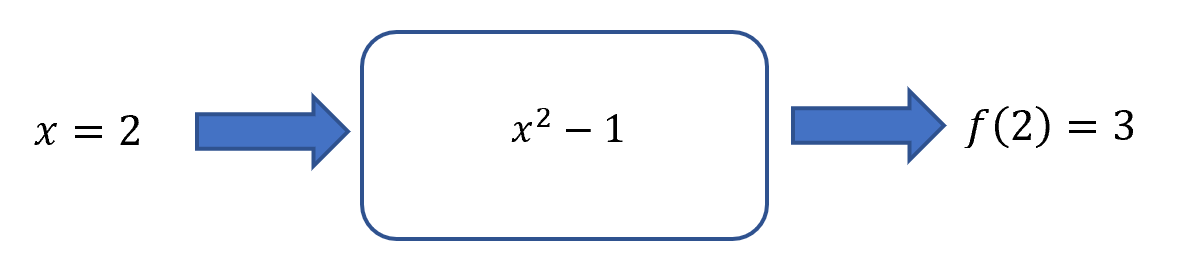• So, when $$x=2, y=3$$ and by using function notation we can write this as $$f(2)=3$$.

## Interval Notation

We know that functions use a set of numbers as input ($$x$$-values) and outputs another set of numbers ($$y$$-values). Now we just need a method to write these sets of numbers.

Interval notation is an easy way of expressing parts of the real number line without having to use variables $$x$$ and $$y$$.

Let’s examine the inequality $$-2<x\leq2$$, which is shown on the number line below: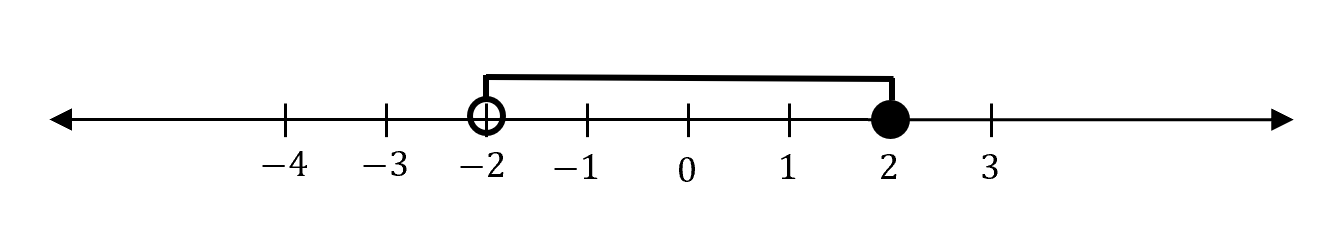To write an interval using interval notation, we use two types of brackets to note the difference between open circles and closed circles.

• $$( )$$ round brackets are used to show the end values is not included.
• $$[ ]$$ square brackets are used to show the end value is included.
• In our example, the interval does not include $$-2$$ (indicated by an open circle), hence we would use the round bracket.
• Furthermore, the interval does include $$2$$ (indicated by a closed circle), hence we would use square brackets.
• Therefore, this interval can be expressed using interval notation as $$(-2,2]$$.

### Intervals to Infinity

We know that number lines go on forever, so we also need a method to write intervals that go on forever. In interval notation, we can express this using the infinity symbol $$\pm\infty$$

Consider the inequality $$x\geq 5$$, shown on the number line below: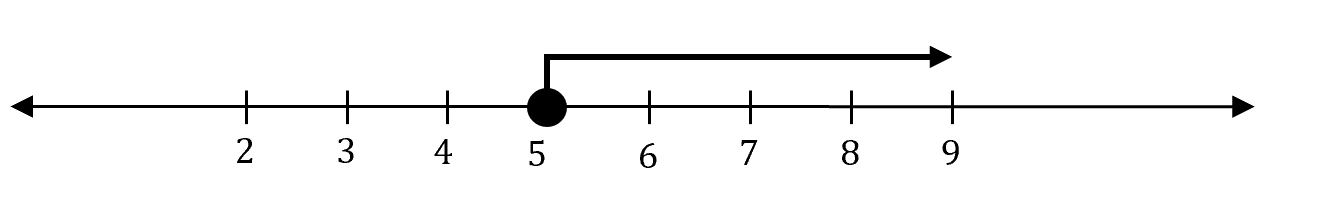This interval can be written as $$[5,\infty)$$.

Note that, we write the infinity symbol with a round bracket and not a square bracket because infinity is not an actual number and hence, we cannot include it on the number line.

### Combining Multiple Intervals

We can also combine multiple intervals using the union symbol $$\cup$$.

Consider the previous two inequalities $$x\geq5$$, $$-2<x\leq2$$.

If we want to express the combination (union) of both these intervals together, we can write it as $$(-2,2] \cup [5,\infty)$$. This is shown on the number line below: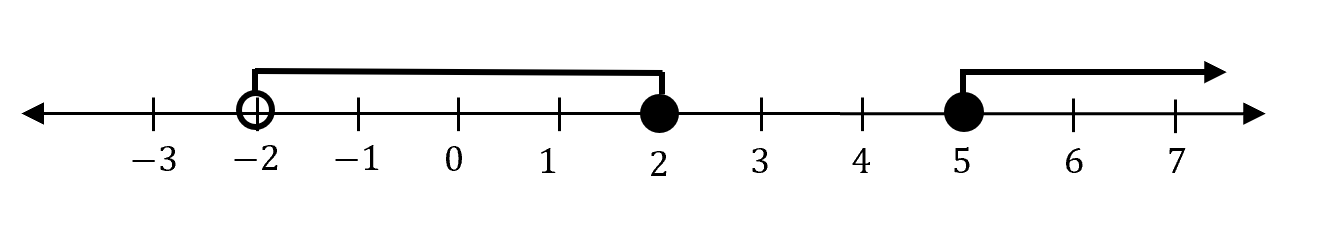## Domain and Range

The set of values that may be assigned to the independent variable $$x$$ is called the domain. It tells us the possible $$x$$-values the relation or function can take.

The set of values that the dependent variable $$y$$ can take is called the range which corresponds to the $$y$$-values.

When writing your solutions, you must state whether you are finding the domain $$(D)$$ or range $$(R)$$.

Let us consider the parabola from our previous example $$y=x^2-1$$.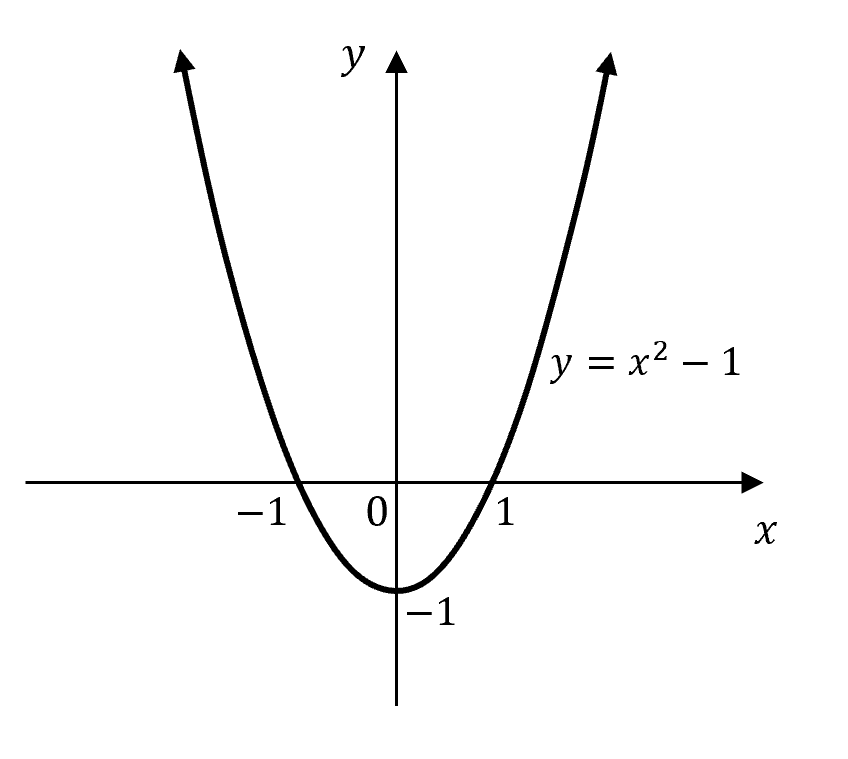By looking at all the $$x$$-values the parabola can take, we can write the domain as $$D=(-\infty,\infty)$$.

Similarly, by looking at all the possible y-values, we can write the range as $$R=[-1,\infty)$$.

## Even and Odd Functions

### Even Functions

A function $$f(x)$$ is called an even function if $$f(-x)= f(x)$$. The graph of an even function is symmetrical about the $$y$$-axis.

Example:

Show that the function $$f(x)=x^2-1$$ is even.

Solution:

To show that a function is even, we need to show that $$f(-x)= f(x)$$.

\begin{align*}
f(-x)&=(-x)^2-1 \\
&=x^2-1 \\
&=f(x)
\end{align*}

So, $$f(-x)= f(x)$$ and the function $$f(x)=x^2-1$$ is even.

### Odd Functions

A function $$f(x)$$ is called an odd function if $$f(-x)= -f(x)$$. The graph of an odd function has 180 degrees point of symmetry about the origin i.e. if you spin your page $$180$$ degrees about the origin, the graph will look the same.

Example:

Show that the function $$f(x)=x^3$$ is odd.

Solution:

To show that a function is even, we need to show that $$f(-x)= -f(x)$$.

\begin{align*}
f(-x)&=(-x)^3 \\
&=-x^3 \\
&=-f(x)
\end{align*}

So, $$f(-x)= -f(x)$$ and the function $$f(x)=x^3$$ is odd.## Concept Check Questions

1. Determine the mapping type for the following sets:

(a)  The set of points $${(1,4),(2,4),(3,5)}$$.

(b)  The set of points $${(a,1),(b,3),(c,3),(c,5)}$$ where $$a$$,$$b$$ and $$c$$ are real numbers.

(c)  The set of points that satisfy the equation $$y=x+1$$.

(d)  The set of points that satisfy the equation $$y=x^2-1$$.

2. Given that $$f(x)=x^2-3x+4$$:

(a)  Evaluate $$f(2)$$.

(b)  Evaluate $$f(-2)$$.

(c)  Evaluate $$f(3)-f(1)$$.

(d)  Find $$f(x+1)-f(x)$$.

3. Express the following intervals using interval notation:

(a)  $$-1<x<0$$.

(b)  $$5\leq \ x<6$$.

(c)  $$x\leq4$$.

(d)  $$x<-2,3\leq \ x\leq6$$.

4. Show that the function:

(a)  $$f(x)=(x^2-3)^3$$ is even.

(b) $$f(x)=x^3-2x$$ is odd.

(c) $$f(x)=(x+1)^2$$ is neither odd nor even.

## Concept Check Solutions

1.

(a)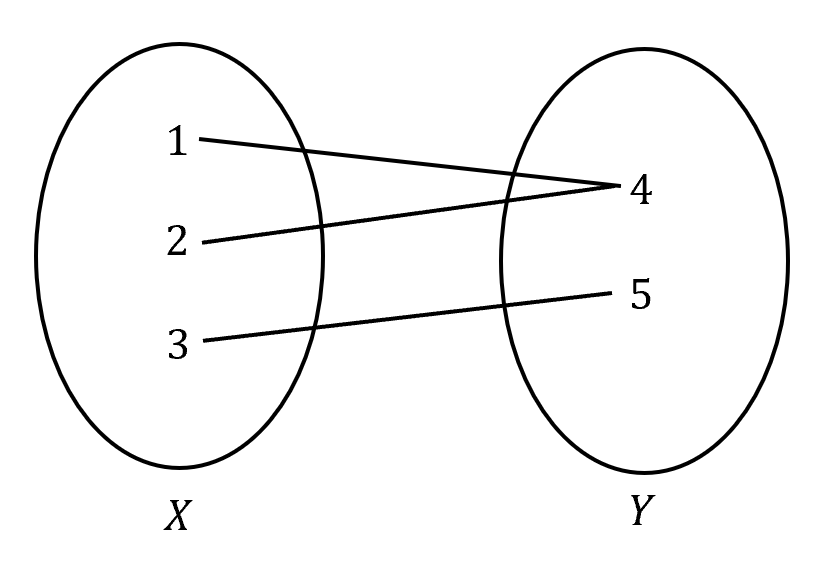many-to-one relation

(b)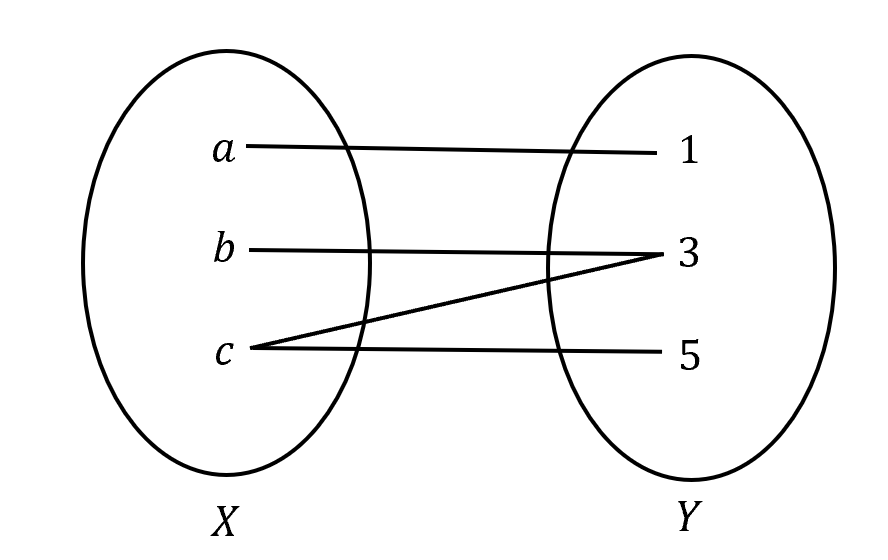many-to-many relation

(c)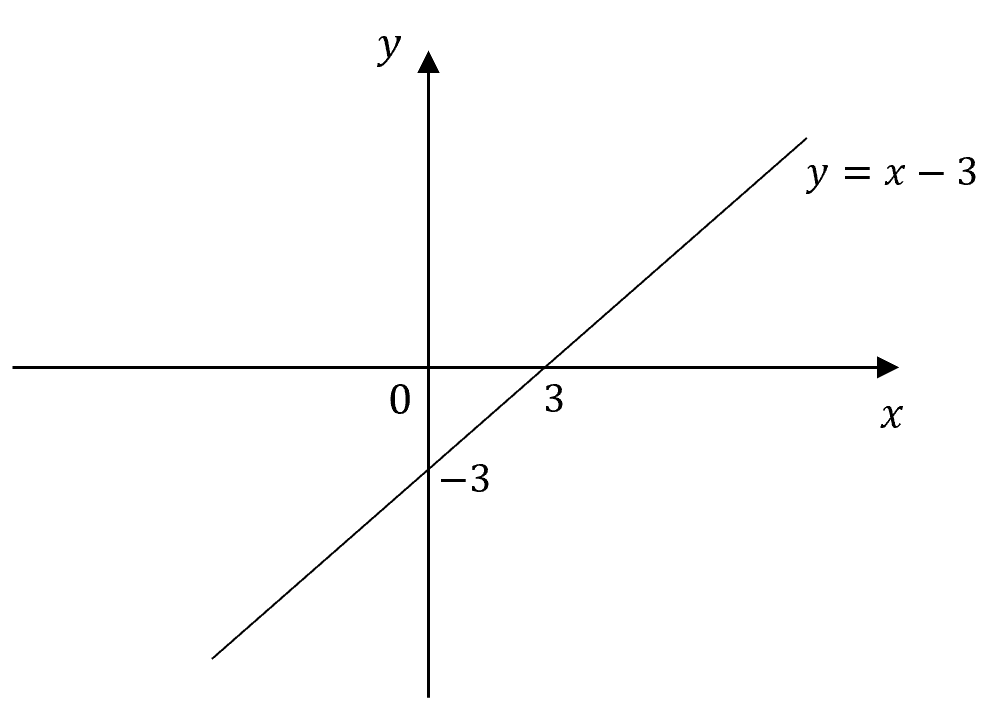one-to-one relation

(d)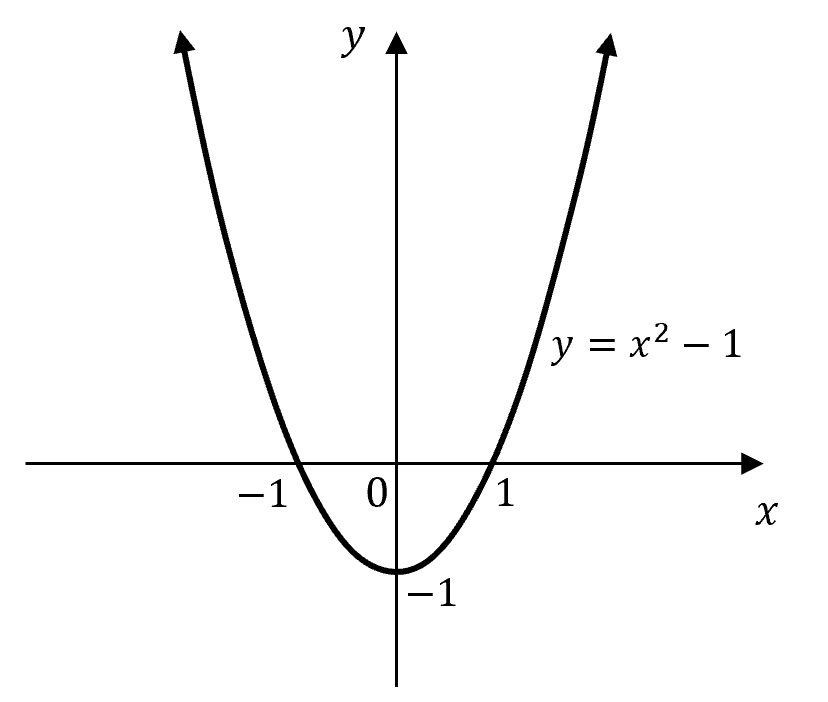many-to-one relation

2.

(a)

\begin{align*}
f(2)&=2^2-3(2)+4\\
&=2\\
\end{align*}

(b)
\begin{align*}
f(-2)&=(-2)^2-3(-2)+4\\
&=14\\
\end{align*}

(c)
\begin{align*}
f(3)-f(1)&=[3^2-3(3)+4]-[1^2-3(1)+4]\\
&=4-2\\
&=2\\
\end{align*}
(d)
\begin{align*}
f(x+1)-f(x)&=[(x+1)^2-3(x+1)+4]-[x^2-3x+4]\\
&=[x^2+2x+1-3x-3+4]-[x^2-3x+4]\\
&=[x^2-x+2]-[x^2-3x+4]\\
&=2x-2\\
\end{align*}
3.

(a)  $$(-1,0)$$

(b)  $$[5,6)$$

(c)  $$(-\infty,4]$$

(d)  $$(-\infty,-2) \cup [3,6]$$

4.

(a)
\begin{align*}
f(-x)&=[(-x)^2-3]^3\\
&=(x^2-3)^3\\
&=f(x)\\
\end{align*}

So, $$f(-x)= f(x)$$ and the function is even.

(b)
\begin{align*}
f(-x)&=(-x)^3-2(-x)\\
&=-x^3+2x=-(x^3-2x)\\
&=-f(x)\\
\end{align*}
So, $$f(-x)= -f(x)$$ and the function is odd.

(c)

 \begin{align*} f(-x)&=(-x+1)^2\\ &=(-1)^2 (x-1)^2\\ &=(x-1)^2\\ \end{align*}

We observe that $$f(-x)\neq f(x)$$ and $$f(-x)\neq -f(x)$$, hence the function is neither odd nor even.

## Want to check your progress with Further Functions and Relations?© Matrix Education and www.matrix.edu.au, 2021. Unauthorised use and/or duplication of this material without express and written permission from this site’s author and/or owner is strictly prohibited. Excerpts and links may be used, provided that full and clear credit is given to Matrix Education and www.matrix.edu.au with appropriate and specific direction to the original content.